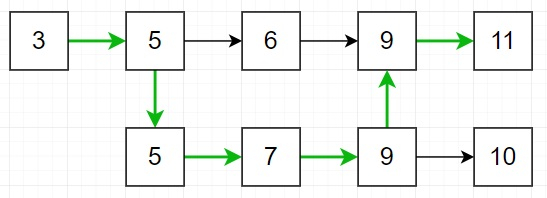# Program to find the maximum score from all possible valid paths in Python

Suppose we have two arrays nums1 and nums2. A valid path is defined as follows −

• Select nums1 or nums2 to traverse (from index-0).

• Traverse the array from left to right.

Now, if we are moving through any value that is present in nums1 and nums2 we can change the path to the other array. Here the score is the sum of unique values in a valid path. We have to find the maximum score we can get of all possible valid paths. If the answer is too large then return result modulo 10^9+7.

So, if the input is like nums1 = [3,5,6,9,11] nums2 = [5,7,9,10], then the output will be 35 because −

• Valid paths starting from nums1 are: [3,5,6,9,11], [3,5,6,9,10], [3,5,7,9,10], [3,5,7,9,11]

• Valid paths starting from nums2 are: [5,7,9,10], [5,6,9,11], [5,6,9,10], [5,7,9,11]So the maximum is [3,5,7,9,11].

To solve this, we will follow these steps −

• M := size of nums1 , N := size of nums2

• sum1 := 0, sum2 := 0

• i := 0, j := 0

• res := 0

• while i < M and j < N, do

• if nums1[i] < nums2[j], then

• sum1 := sum1 + nums1[i]

• i := i + 1

• otherwise when nums1[i] > nums2[j], then

• sum2 := sum2 + nums2[j]

• j := j + 1

• otherwise,

• res := res + maximum of sum1, (sum2 + nums1[i])

• i := i + 1

• j := j + 1

• sum1 := 0

• sum2 := 0

• while i < M , do

• sum1 := sum1 + nums1[i]

• i := i + 1

• while j < N , do

• sum2 := sum2 + nums2[j]

• j := j + 1

• return(res + maximum of sum1, sum2) mod 10^9+7

## Example

Let us see the following implementation to get better understanding

def solve(nums1, nums2):
M, N = len(nums1), len(nums2)
sum1, sum2 = 0, 0
i, j = 0, 0
res = 0
while i < M and j < N:
if nums1[i] < nums2[j]:
sum1 += nums1[i]
i += 1
elif nums1[i] > nums2[j]:
sum2 += nums2[j]
j += 1
else:
res += max(sum1, sum2) + nums1[i]
i += 1
j += 1
sum1 = 0
sum2 = 0

while i < M:
sum1 += nums1[i]
i += 1
while j < N:
sum2 += nums2[j]
j += 1
return (res + max(sum1, sum2)) % 1000000007

nums1 = [3,5,6,9,11]
nums2 = [5,7,9,10]
print(solve(nums1, nums2))

## Input

[3,5,6,9,11], [5,7,9,10]


## Output

35